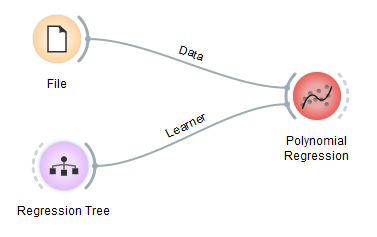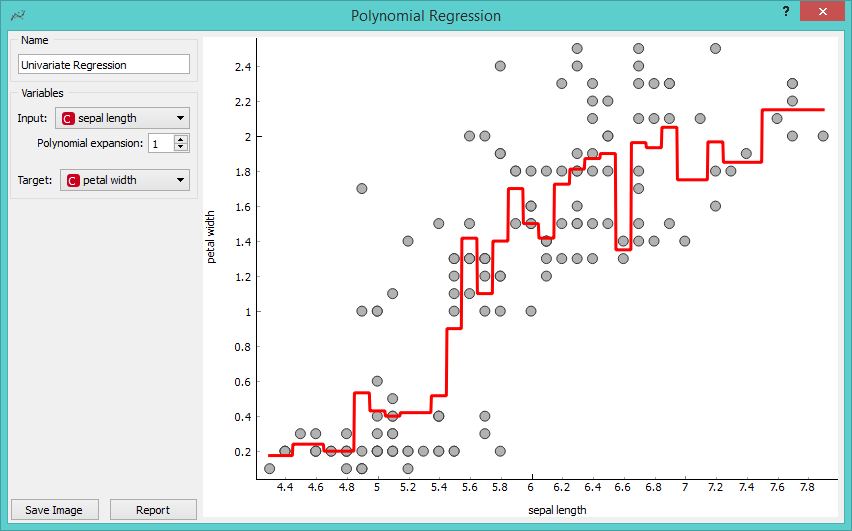# Polynomial Regression¶Educational widget that interactively shows regression line for different regressors.

## Signals¶

Inputs:

• Data

Input data set. It needs at least two continuous attributes.

• Preprocessor

Data preprocessors.

• Learner

Regression algorithm used in the widget. Default set to Linear Regression.

Outputs:

• Learner

Regression algorithm used in the widget.

• Predictor

Trained regressor.

• Coefficients

Regressor coefficients if it has them.

## Description¶

This widget interactively shows regression line using any of the regressors from Orange3 Regression module. In the widget, polynomial expansion can be set. Polynomial expansion is a regulation of the degree of polynom that is used to transform the input data and has an effect on the shape of a curve. If polynomial expansion is set to 1 it means that untransformed data are used in the regression.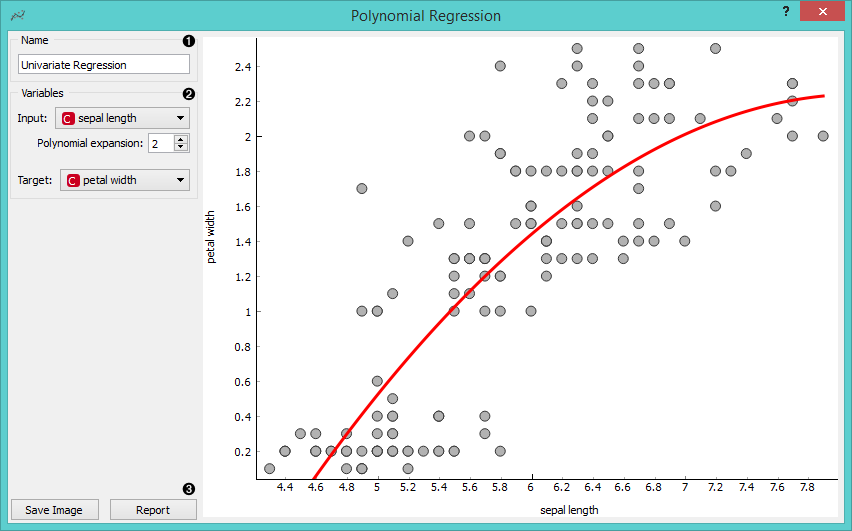1. Regressor name.

2. Input: independent variable on axis x.

Polynomial expansion: degree of polynomial expansion.

Target: dependent variable on axis y.

3. Save Image saves the image to the computer in a .svg or .png format.

Report includes widget parameters and visualization in the report.

## Example¶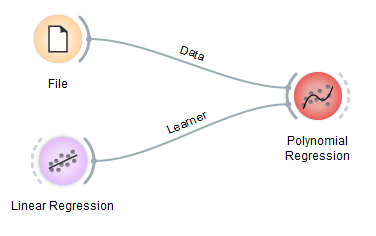We loaded iris data set with the File widget. Then we connected Linear Regression learner to the Polynomial Regression widget. In the widget we selected petal length as our Input variable and petal width as our Target variable. We set Polynomial expansion to 1 which gives us a linear regression line. The result is shown on the figure below.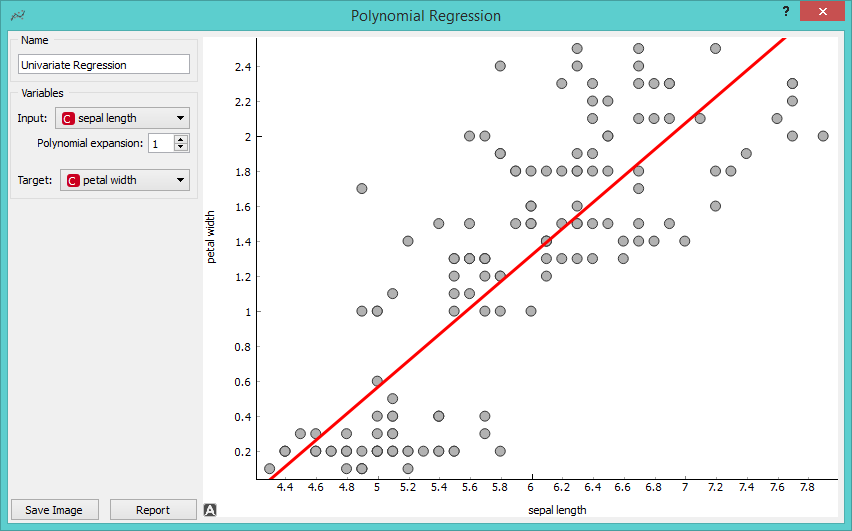The line can fit better if we increase the Polynomial expansion parameter. Say, we set it to 3.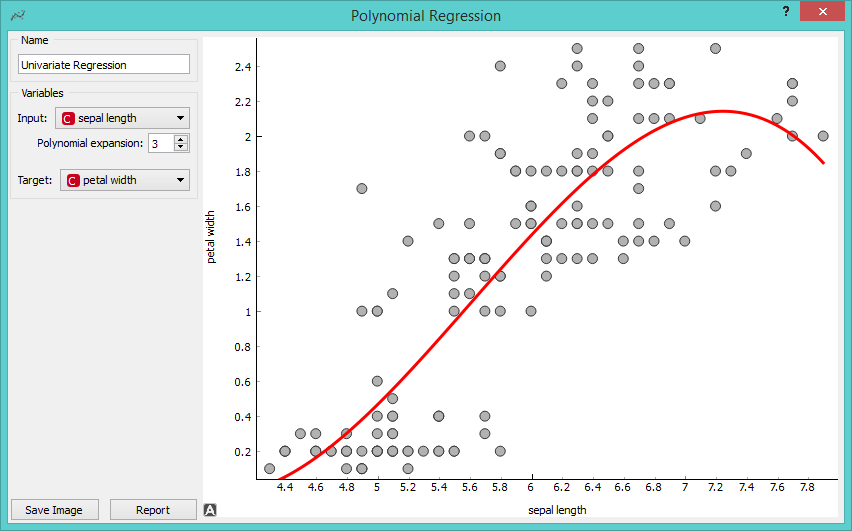To observe different results, change Linear Regression to any other regression learner from Orange. Example below is done with Regression Tree learner.